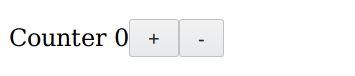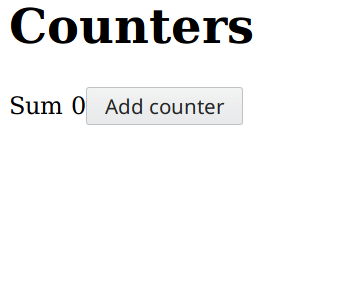Package

# purescript-turbine

Repository
funkia/purescript-turbine
MIT
paldepind
Published on
2019-02-25

• Concise and powerful thanks to FRP.
• No big global state/model. Everything is incapsulated in components.
• Type-safe communication between views and models.
• Model logic and view code is kept seperate for logic-less views.

## Examples

### Single counter```counterModel {increment, decrement} init = do
let changes = (increment \$> 1) <> (decrement \$> -1)
count <- sample \$ scan (+) init changes
pure {count}

counterView {count} =
E.text "Counter " </>
E.span (E.textB \$ map show count) </>
E.button "+" `output` (\o -> {increment: o.click}) </>
E.button "-" `output` (\o -> {decrement: o.click})

counter = modelView counterModel counterView

main = runComponent "#mount" (counter 0)```

### List of counters

Show a list of counters. New counters can be added to the list. Existing counters can be deleted. The aggregated sum of all the counters is shown.```counterModel {increment, decrement, delete} id = do
let changes = (increment \$> 1) <> (decrement \$> -1)
count <- sample \$ scan (+) 0 changes
pure {count, delete: delete \$> id}

counterView {count} =
E.div (
E.text "Counter" </>
E.span (E.textB \$ map show count) </>
E.button "+" `output` (\o -> {increment: o.click}) </>
E.button "-" `output` (\o -> {decrement: o.click}) </>
E.button "x" `output` (\o -> {delete: o.click})
)

counter = modelView counterModel counterView

counterListModel {addCounter, listOut} init = do
let sum = listOut >>= (map (_.count) >>> foldr (lift2 (+)) (pure 0))

let removeId = map (fold <<< map (_.delete)) listOut
let removeCounter = map (\i -> filter (i /= _)) (switchStream removeId)

nextId <- sample \$ scanS (+) 0 (addCounter \$> 1)
let appendCounter = cons <\$> nextId

counterIds <- sample \$ scan (\$) init (appendCounter <> removeCounter)
pure {sum, counterIds}

counterListView {sum, counterIds} =
E.div (
E.h1 (E.text "Counters") </>
E.span (E.textB (map (\n -> "Sum " <> show n) sum)) </>
list counter counterIds id `output` (\o -> {listOut: o})
)

counterList = modelView counterListModel counterListView

main = runComponent "#mount" (counterList )```

## Installation

The following installs Hareactive and Turbine. Hareactive is the FRP library that Turbine builds upon and is a hard dependency.

``````npm i @funkia/hareactive
bower install --save purescript-hareactive
npm i @funkia/turbine
bower install --save purescript-turbine
``````

## Tutorial

This is a hands-on tutorial in which we build a simple application. The core concepts in Turbine are introduced along the way. Turbine is based on functional reactive programming (FRP). In particular it uses the FRP library Hareactive. This tutorial assumes no prior experience with FRP and hence it can also be seen as an introduction to FRP.

If you want to you can follow along the tutorial yourself. You can do so by cloning the Turbine starter template.

``````git clone https://github.com/funkia/purescript-turbine-starter turbine-tutorial
cd turbine-tutorial
npm i
``````

You can then run `npm run build` and aftewards you should see the text "Hello, world!" if you open the `index.html` file in a browser. Along the way you should make changes to the file `src/Main.purs`.

### Component

The central type in Turbine is `Component`. A `Component` represents a piece of user interface. For instance, that could be an input field or a button. More concretely a `Component` is a description on how to create a piece of HTML. Components are composable. Hence an input field and a button can be composed together and the result is another component. A Turbine application is "components all the way down".

The `Component` type has the following kind.

``````Component :: Type -> Type -> Type
``````

That is, it is parameterized by two types. The purpose of those are explained later.

### Creating simple HTML

Turbine contains functions for creating components that correspond to single HTML elements. These live in the module `Turbine.HTML.Elements` which is typically import qualified like this.

`import Turbine.HTML.Elements as E`

For each HTML element the module exports a corresponding function. For the HTML element `div` there is a function `div`, for the `span` element there is a `span` function, and so on. The first argument to these functions is a record of attributes. If the HTML element supports children then the corresponding function takes a second argument as well which is a component. Here are a few examples.

```myInput = E.input { placeholder: "Write here", class: "form-input" }
myButton = E.button {} (E.text "Click me")
myDivWithButton = E.div { class: "div-class" } myButton```

The `text` function used above takes a string an returns a component corressponding to a text node of the string.

Components are composed together with the `</>` operator. As a first approximation `</>` is similar to the semigroup operator `<>`. However, the type of `</>` is slightly different as we will see later. Writing `component1 </> component2` creates a new component which represents the HTML from the first component followed by the HTML for the second component. Here is an example.

```const myLoginForm =
E.input { placeholder: "Username" } </>
E.input { placeholder: "Password" } </>
E.label {} (E.text "Remember login") </>
E.input { type: "checkbox" }```

If you add the following to the code in `Main.purs` and change `Main` into the following.

```-app = E.text "Hello, world!"

Then you should see HTML corresponding to the following.

```<input placeholder="Username" />
<input type="checkbox" />```

By combining `</>` with the fact that the element function accept a child component as their second argument we can create arbitrary HTML. Now, let us create the HTML which we will use going forward.

```counterView =
E.div {} (
E.text "Counter " </>
E.span {} (E.text "0") </>
E.button {} (E.text "+") </>
E.button {} (E.text "-")
)```

Here we have hardcoded the value `0` into the user interface. The intended outcome is that the displayed number is dynamic and increments every time the `+` button is pressed and decrements every time the `-` button is pressed. But, before we can implement that we need to learn a little bit of FRP.

### A short interlude on FRP

Functional reactive programming contains two key data-types `Behavior` and `Stream`.

Note: What we call `Stream` is often called `Event` in other FRP libraries.

A `Behavior` represents a value that changes over time. For instance, `Behavior Number` represents a changing number and `Behavior String` represents a changing string.

A `Stream` represents events or occurrences that happens at specific moments in time.

The difference between behaviors and streams can be illustrated as below.As the image indicates a behavior can be seen a function from time. That is, at any specific moment in time it has a value. A stream on the other hand only has values, or occurrences, at specific punctuations in time.

### Dynamic HTML

In the counter component above we hard-coded the value `0` into the view. The goal is that the displayed number should change over time. And, as mentioned, in FRP we use behaviors to represent values that change over time. Thus, we parametize the HTML above such that it takes as agument a record of a behavior of the type `Behavior Number`.

```counterView { count } =
E.div {} (
E.text "Counter " </>
E.span {} (E.textB (map show count)) </>
E.button {} (E.text "+") </>
E.button {} (E.text "-")
)```

We also changed `E.text "0"` into `E.textB (map show count)`. The `textB` function is similar to `text` except that instead of taking an argument of type `String` it takes an argument of type `Behavior String.` It then returns a component that describes dynamic HTML. At any point in time the value of the text node will have the same value as the behavior.

### Output

Recall that the `Component` type is parameterized by two types. The first of these is called the components selected output and the second is called the available output.